# http www comp nus edu sgcs 1010 UNIT

• Slides: 44http: //www. comp. nus. edu. sg/~cs 1010/ UNIT 5 Top-Down Design & FunctionsAaron Tan, NUS Top-Down Design & Functions Unit 5 - 2 Unit 5: Top-Down Design & Functions Objectives: § How to analyse, design, and implement a § § program How to break a problem into sub-problems with step-wise refinement How to create your own user-defined functions Reference: § Chapter 3 Top-Down Design with FunctionsAaron Tan, NUS Top-Down Design & Functions Unit 5 - 3 Unit 4: Top-Down Design & Functions (1/2) 1. Problem Solving 2. Case Study: Top-Down Design § Computing the weight of a batch of flat washers § Incremental Refinement (some hierarchical chart) § Top-down design (of program) with structure charts 3. Function Prototypes 4. Default Return Type 5. ‘return’ statement in main()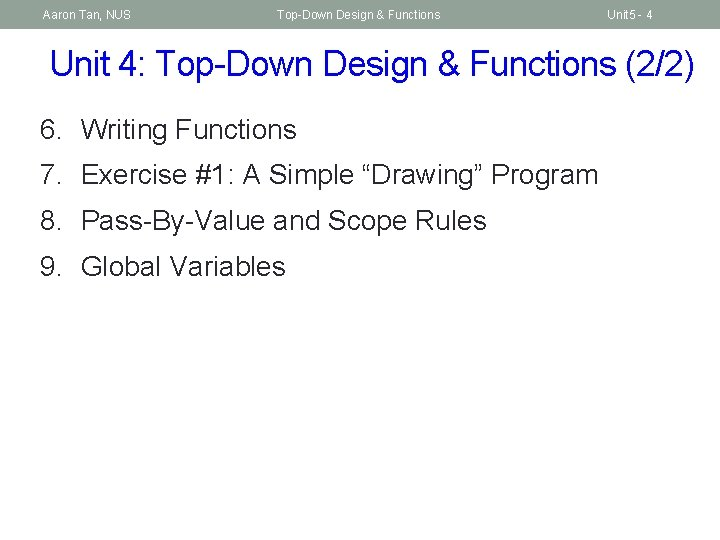Aaron Tan, NUS Top-Down Design & Functions Unit 5 - 4 Unit 4: Top-Down Design & Functions (2/2) 6. Writing Functions 7. Exercise #1: A Simple “Drawing” Program 8. Pass-By-Value and Scope Rules 9. Global Variables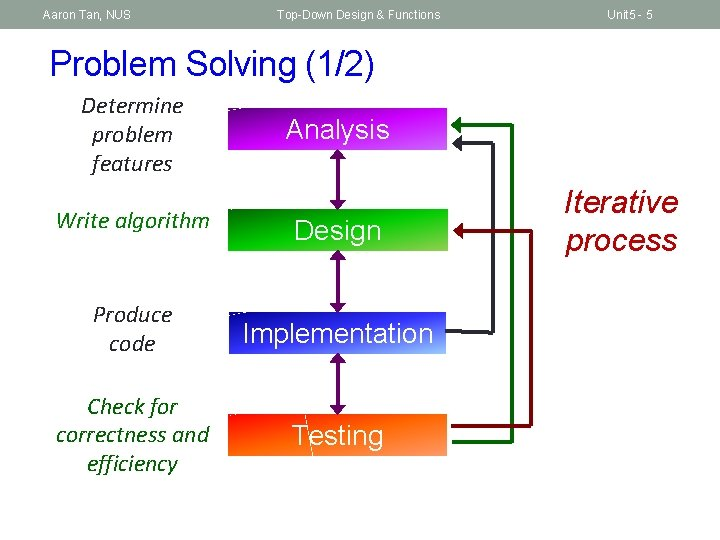Aaron Tan, NUS Top-Down Design & Functions Unit 5 - 5 Problem Solving (1/2) Determine problem features Analysis Write algorithm Design Produce code Implementation Check for correctness and efficiency Testing Iterative processAaron Tan, NUS Top-Down Design & Functions Unit 5 - 6 Problem Solving (2/2) Analysis and Design stepwise refinement NO (hierarchy of) sub-problems Can sub-problems be implemented? Knowledge in C and its libraries Knowledge in algorithms YES structure chart Knowledge in data structures (mostly CS 2040) Implementation & Testing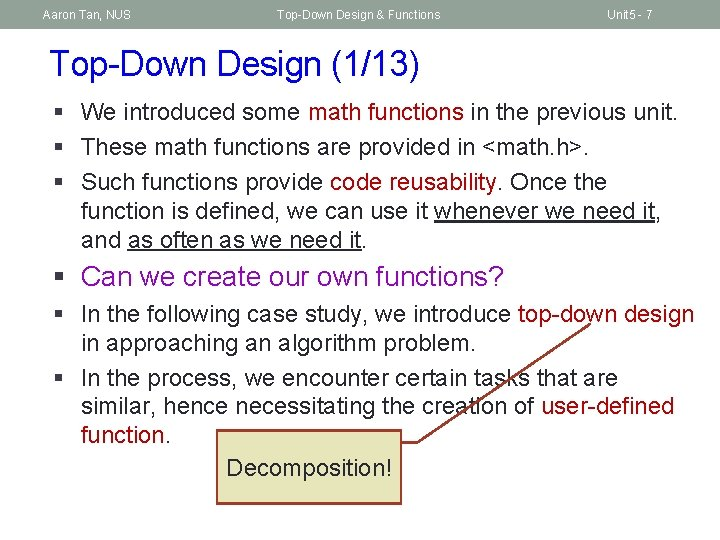Aaron Tan, NUS Top-Down Design & Functions Unit 5 - 7 Top-Down Design (1/13) § We introduced some math functions in the previous unit. § These math functions are provided in <math. h>. § Such functions provide code reusability. Once the function is defined, we can use it whenever we need it, and as often as we need it. § Can we create our own functions? § In the following case study, we introduce top-down design in approaching an algorithm problem. § In the process, we encounter certain tasks that are similar, hence necessitating the creation of user-defined function. Decomposition!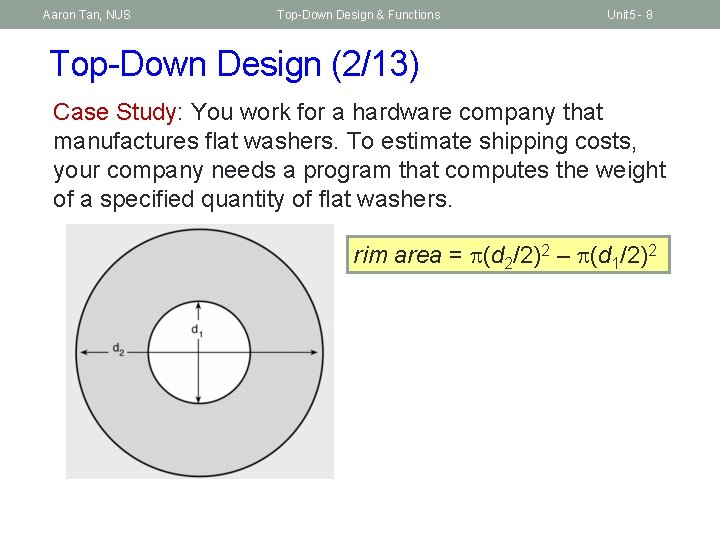Aaron Tan, NUS Top-Down Design & Functions Unit 5 - 8 Top-Down Design (2/13) Case Study: You work for a hardware company that manufactures flat washers. To estimate shipping costs, your company needs a program that computes the weight of a specified quantity of flat washers. rim area = (d 2/2)2 – (d 1/2)2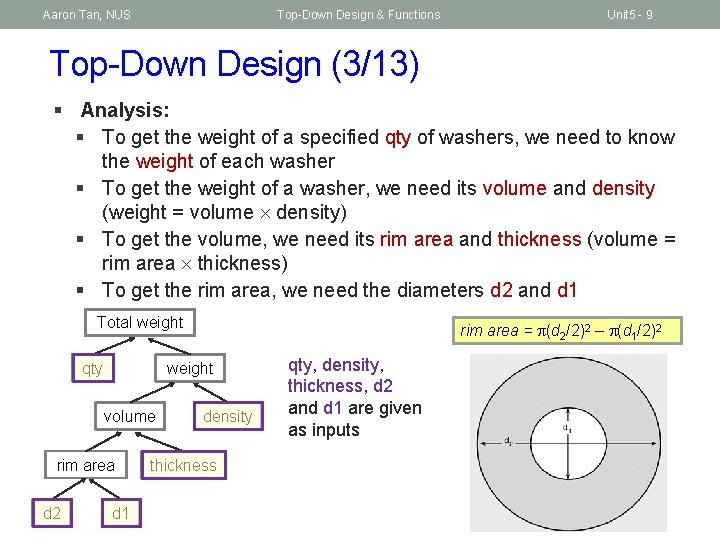Aaron Tan, NUS Top-Down Design & Functions Unit 5 - 9 Top-Down Design (3/13) § Analysis: § To get the weight of a specified qty of washers, we need to know the weight of each washer § To get the weight of a washer, we need its volume and density (weight = volume density) § To get the volume, we need its rim area and thickness (volume = rim area thickness) § To get the rim area, we need the diameters d 2 and d 1 Total weight qty weight volume rim area d 2 rim area = (d 2/2)2 – (d 1/2)2 d 1 density thickness qty, density, thickness, d 2 and d 1 are given as inputs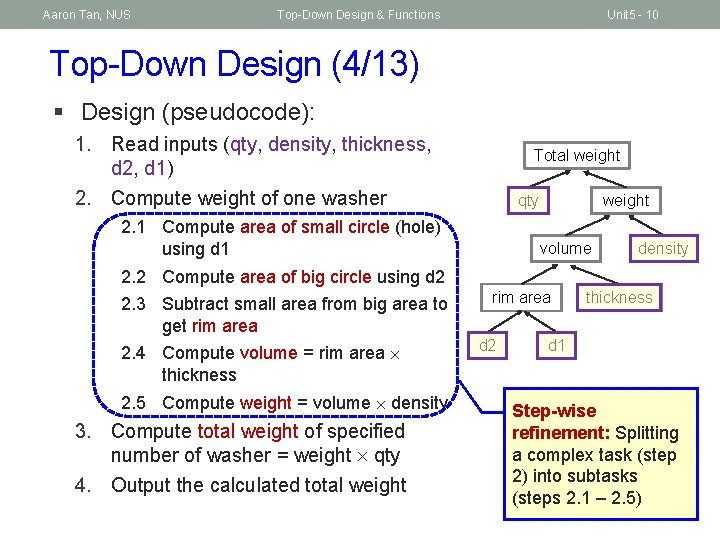Aaron Tan, NUS Top-Down Design & Functions Unit 5 - 10 Top-Down Design (4/13) § Design (pseudocode): 1. Read inputs (qty, density, thickness, d 2, d 1) 2. Compute weight of one washer Total weight qty 2. 1 Compute area of small circle (hole) using d 1 weight volume density 2. 2 Compute area of big circle using d 2 2. 3 Subtract small area from big area to get rim area 2. 4 Compute volume = rim area thickness 2. 5 Compute weight = volume density 3. Compute total weight of specified number of washer = weight qty 4. Output the calculated total weight rim area d 2 thickness d 1 Step-wise refinement: Splitting a complex task (step 2) into subtasks (steps 2. 1 – 2. 5)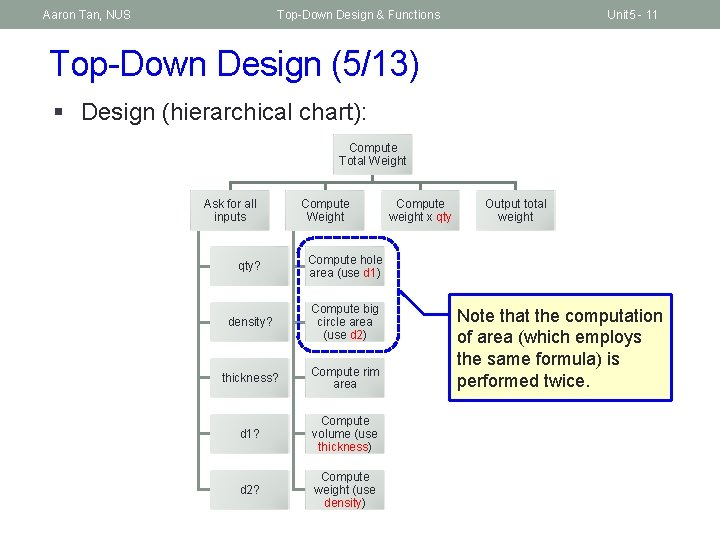Aaron Tan, NUS Top-Down Design & Functions Unit 5 - 11 Top-Down Design (5/13) § Design (hierarchical chart): Compute Total Weight Ask for all inputs Compute Weight qty? Compute hole area (use d 1) density? Compute big circle area (use d 2) thickness? Compute rim area d 1? Compute volume (use thickness) d 2? Compute weight (use density) Compute weight x qty Output total weight Note that the computation of area (which employs the same formula) is performed twice.Aaron Tan, NUS Top-Down Design & Functions Unit 5 - 12 Top-Down Design (6/13) § Design (structure chart): § A documentation tool that shows the relationship among the subtasks Compute Total Weight Input : qty, density, thickness, d 1, d 2 Compute Weight of a single washer Compute circle area Compute total Weight Output total weight This is called twice.Aaron Tan, NUS Top-Down Design & Functions Unit 5 - 13 Top-Down Design (7/13) Unit 5_Washers. c #include <stdio. h> #include <math. h> #define PI 3. 14159 int main(void) { double d 1, d 2, thickness, density; int qty; double unit_weight, total_weight, outer_area, inner_area, rim_area; // hole circle diameter // big circle diameter // // // single washer's weight a batch of washers' total weight area of big circle area of small circle single washer's rim area // read input data printf("Inner diameter in cm: "); scanf("%lf", &d 1); printf("Outer diameter in cm: "); scanf("%lf", &d 2); printf("Thickness in cm: "); scanf("%lf", &thickness); printf("Density in grams per cubic cm: "); scanf("%lf", &density); printf("Quantity: "); scanf("%d", &qty);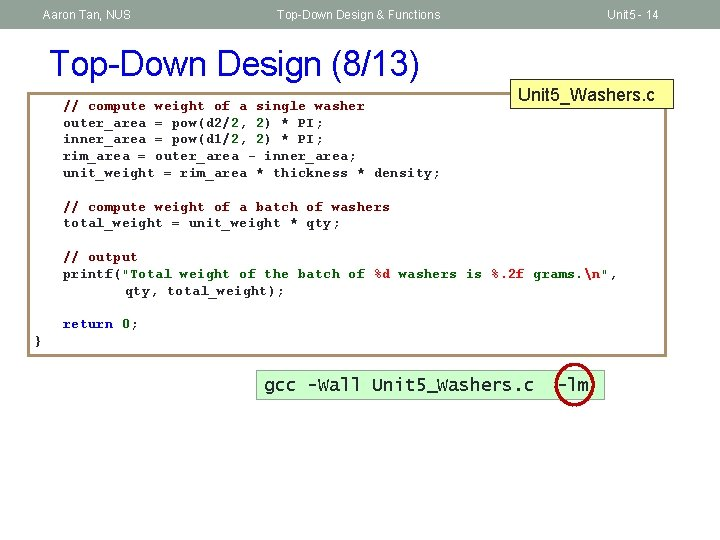Aaron Tan, NUS Top-Down Design & Functions Unit 5 - 14 Top-Down Design (8/13) // compute weight of a single washer outer_area = pow(d 2/2, 2) * PI; inner_area = pow(d 1/2, 2) * PI; rim_area = outer_area - inner_area; unit_weight = rim_area * thickness * density; Unit 5_Washers. c // compute weight of a batch of washers total_weight = unit_weight * qty; // output printf("Total weight of the batch of %d washers is %. 2 f grams. n", qty, total_weight); return 0; } gcc -Wall Unit 5_Washers. c -lmAaron Tan, NUS Top-Down Design & Functions Unit 5 - 15 Top-Down Design (9/13) § Note that area of circle is computed twice. For code reusability, it is better to define a function to compute area of a circle. double circle_area(double diameter) { return pow(diameter/2, 2) * PI; } § We can then call/invoke this function whenever we need it. circle_area(d 2) to compute area of circle with diameter d 2 circle_area(d 1) to compute area of circle with diameter d 1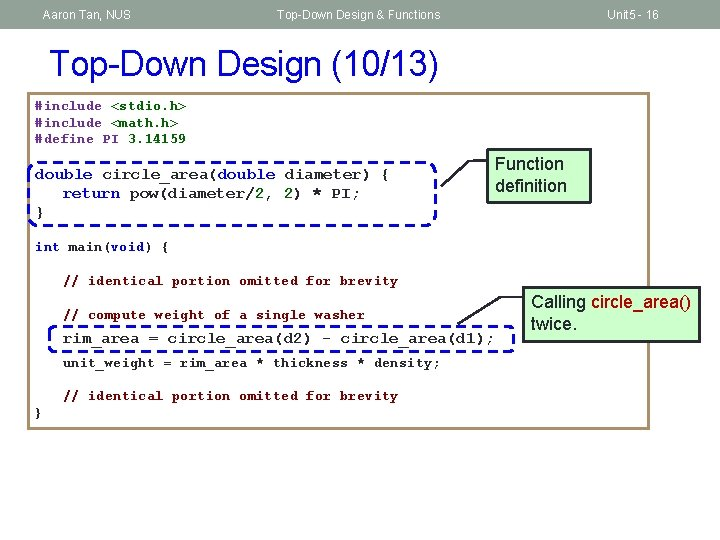Aaron Tan, NUS Top-Down Design & Functions Unit 5 - 16 Top-Down Design (10/13) #include <stdio. h> #include <math. h> #define PI 3. 14159 double circle_area(double diameter) { return pow(diameter/2, 2) * PI; } Function definition int main(void) { // identical portion omitted for brevity // compute weight of a single washer rim_area = circle_area(d 2) - circle_area(d 1); unit_weight = rim_area * thickness * density; // identical portion omitted for brevity } Calling circle_area() twice.Aaron Tan, NUS Top-Down Design & Functions Unit 5 - 17 Top-Down Design (11/13) § Components of a function definition § Header (or signature): consists of return type, function name, and a list of parameters (with their types) separated by commas § Function names follow identifier rules (just like variable names) § May consist of letters, digit characters, or underscore, but cannot begin with a digit character § Return type is void if function does not need to return any value § Function body: code to perform the task; contains a return statement if return type is not void Return type Function name double circle_area(double diameter) { return pow(diameter/2, 2) * PI; } Parameter Function body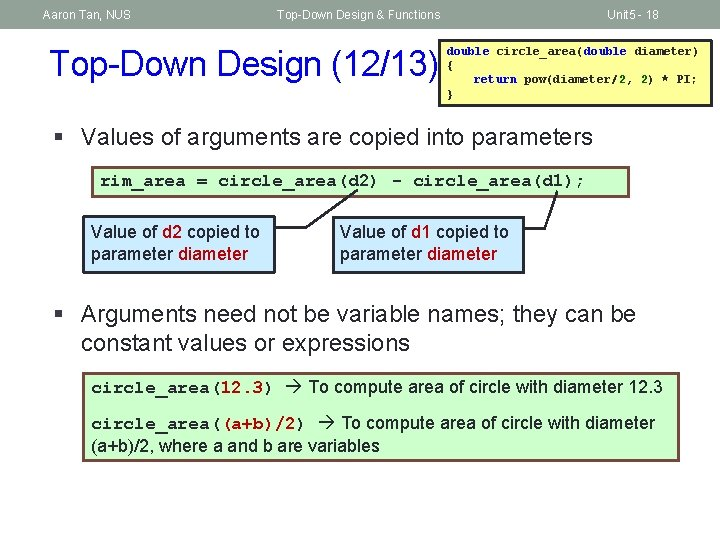Aaron Tan, NUS Top-Down Design & Functions Top-Down Design (12/13) Unit 5 - 18 double circle_area(double diameter) { return pow(diameter/2, 2) * PI; } § Values of arguments are copied into parameters rim_area = circle_area(d 2) - circle_area(d 1); Value of d 2 copied to parameter diameter Value of d 1 copied to parameter diameter § Arguments need not be variable names; they can be constant values or expressions circle_area(12. 3) To compute area of circle with diameter 12. 3 circle_area((a+b)/2) To compute area of circle with diameter (a+b)/2, where a and b are variables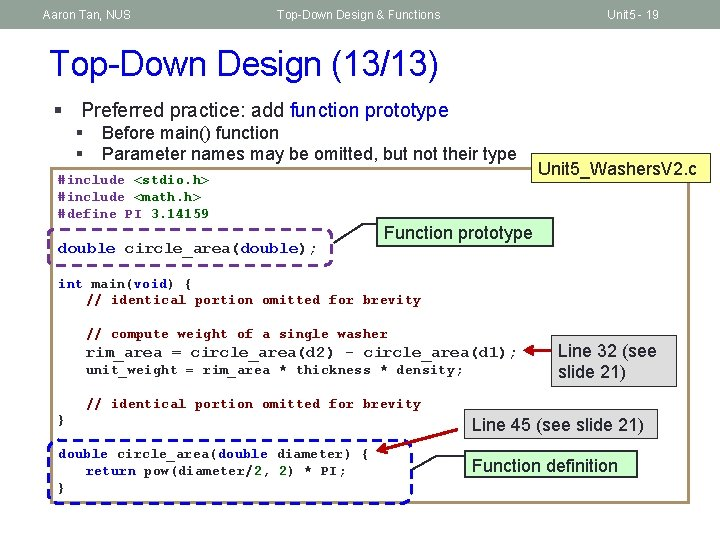Aaron Tan, NUS Top-Down Design & Functions Unit 5 - 19 Top-Down Design (13/13) § Preferred practice: add function prototype § § Before main() function Parameter names may be omitted, but not their type #include <stdio. h> #include <math. h> #define PI 3. 14159 double circle_area(double); Unit 5_Washers. V 2. c Function prototype int main(void) { // identical portion omitted for brevity // compute weight of a single washer rim_area = circle_area(d 2) - circle_area(d 1); unit_weight = rim_area * thickness * density; Line 32 (see slide 21) // identical portion omitted for brevity } Line 45 (see slide 21) double circle_area(double diameter) { return pow(diameter/2, 2) * PI; } Function definition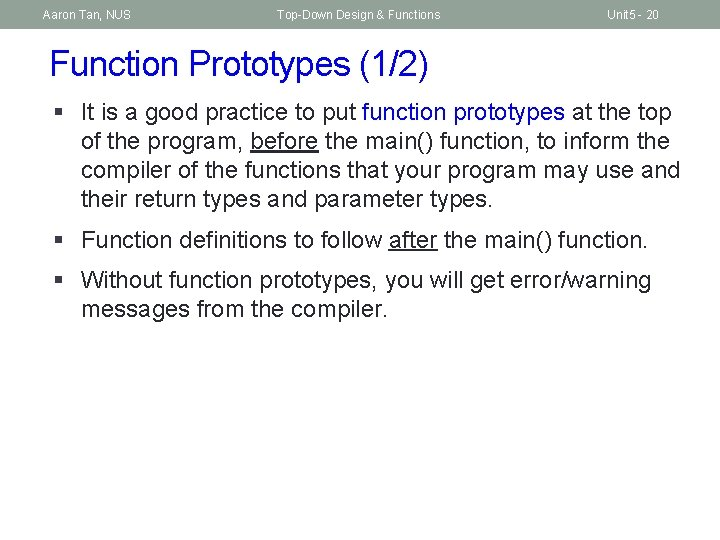Aaron Tan, NUS Top-Down Design & Functions Unit 5 - 20 Function Prototypes (1/2) § It is a good practice to put function prototypes at the top of the program, before the main() function, to inform the compiler of the functions that your program may use and their return types and parameter types. § Function definitions to follow after the main() function. § Without function prototypes, you will get error/warning messages from the compiler.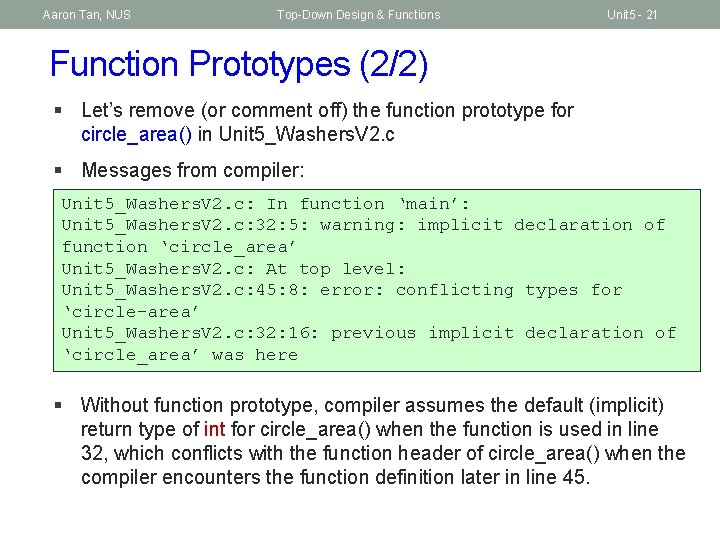Aaron Tan, NUS Top-Down Design & Functions Unit 5 - 21 Function Prototypes (2/2) § Let’s remove (or comment off) the function prototype for circle_area() in Unit 5_Washers. V 2. c § Messages from compiler: Unit 5_Washers. V 2. c: In function ‘main’: Unit 5_Washers. V 2. c: 32: 5: warning: implicit declaration of function ‘circle_area’ Unit 5_Washers. V 2. c: At top level: Unit 5_Washers. V 2. c: 45: 8: error: conflicting types for ‘circle-area’ Unit 5_Washers. V 2. c: 32: 16: previous implicit declaration of ‘circle_area’ was here § Without function prototype, compiler assumes the default (implicit) return type of int for circle_area() when the function is used in line 32, which conflicts with the function header of circle_area() when the compiler encounters the function definition later in line 45.Aaron Tan, NUS Top-Down Design & Functions Unit 5 - 22 Default Return Type (1/3) § A ‘type-less’ function has default return type of int 1 2 3 4 5 6 7 8 9 10 #include <stdio. h> int main(void) { printf("%dn", f(100, 7)); return 0; } f(int a, int b) { return a*b*b; } § Program can be compiled, but with warning: implicit declaration of function 'f' line 4 (due to absence of function prototype) warning: return type defaults to 'int' line 8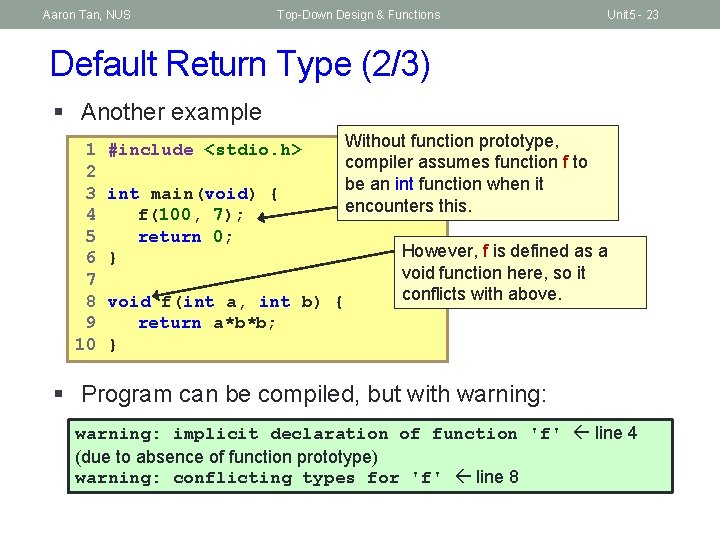Aaron Tan, NUS Top-Down Design & Functions Unit 5 - 23 Default Return Type (2/3) § Another example 1 2 3 4 5 6 7 8 9 10 #include <stdio. h> int main(void) { f(100, 7); return 0; } void f(int a, int b) { return a*b*b; } Without function prototype, compiler assumes function f to be an int function when it encounters this. However, f is defined as a void function here, so it conflicts with above. § Program can be compiled, but with warning: implicit declaration of function 'f' line 4 (due to absence of function prototype) warning: conflicting types for 'f' line 8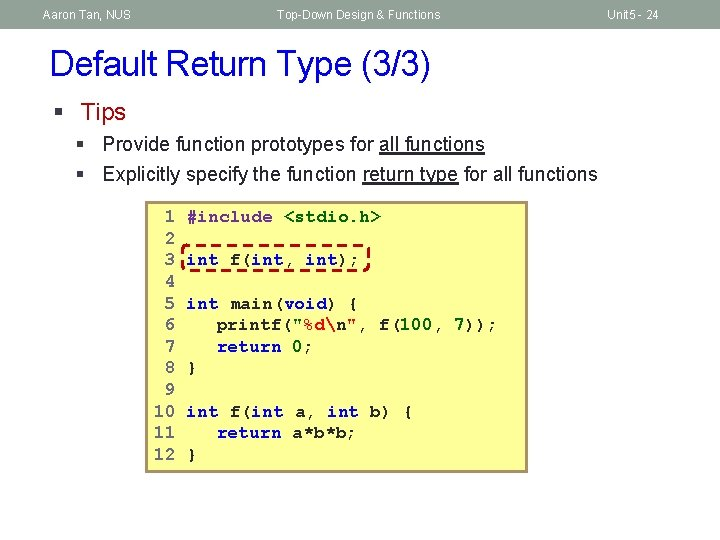Aaron Tan, NUS Top-Down Design & Functions Default Return Type (3/3) § Tips § Provide function prototypes for all functions § Explicitly specify the function return type for all functions 1 2 3 4 5 6 7 8 9 10 11 12 #include <stdio. h> int f(int, int); int main(void) { printf("%dn", f(100, 7)); return 0; } int f(int a, int b) { return a*b*b; } Unit 5 - 24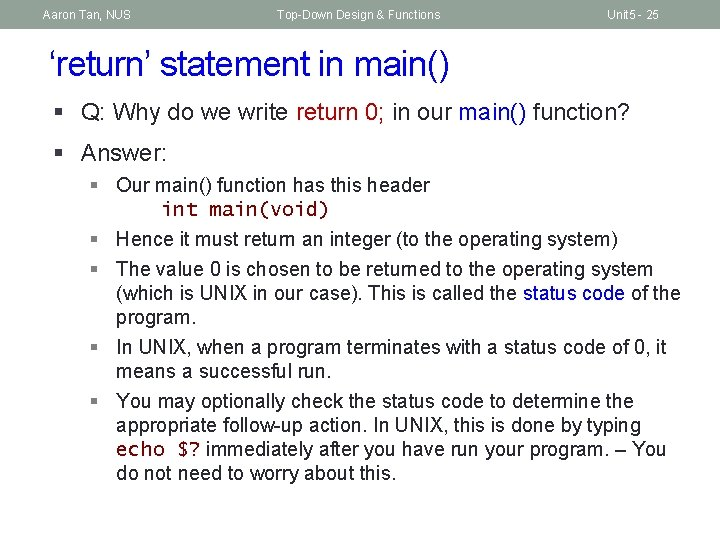Aaron Tan, NUS Top-Down Design & Functions Unit 5 - 25 ‘return’ statement in main() § Q: Why do we write return 0; in our main() function? § Answer: § Our main() function has this header int main(void) § Hence it must return an integer (to the operating system) § The value 0 is chosen to be returned to the operating system (which is UNIX in our case). This is called the status code of the program. § In UNIX, when a program terminates with a status code of 0, it means a successful run. § You may optionally check the status code to determine the appropriate follow-up action. In UNIX, this is done by typing echo \$? immediately after you have run your program. – You do not need to worry about this.Aaron Tan, NUS Top-Down Design & Functions Unit 5 - 26 Writing Functions (1/5) § A program is a collection of functions (modules) to transform inputs to outputs § In general, each box in a structure chart is a sub-problem which is handled by a function § In mathematics, a function maps some input values to a single (possibly multiple dimensions) output § In C, a function maps some input values to zero or more output values § No output: void func(…) { … } § One output, e. g. , double func(…) { …; return value; } § More outputs through changing input values (we’ll cover this later) § Return value (if any) from function call can (but need not) be assigned to a variable.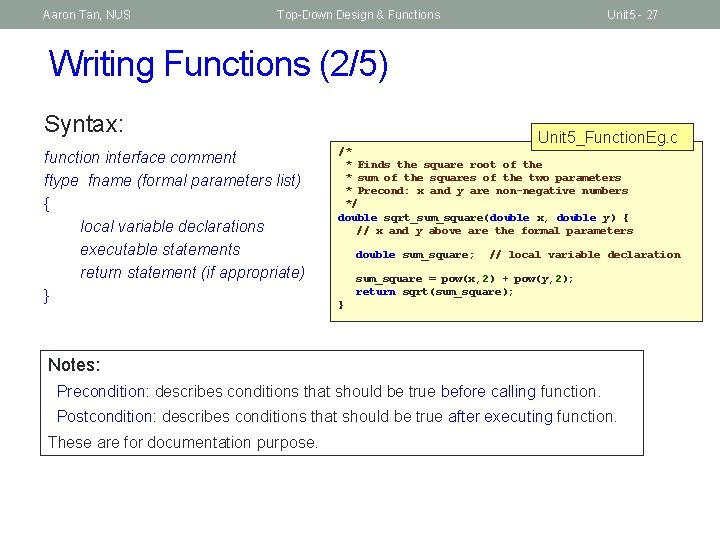Aaron Tan, NUS Top-Down Design & Functions Unit 5 - 27 Writing Functions (2/5) Syntax: function interface comment ftype fname (formal parameters list) { local variable declarations executable statements return statement (if appropriate) } Unit 5_Function. Eg. c /* * Finds the square root of the * sum of the squares of the two parameters * Precond: x and y are non-negative numbers */ double sqrt_sum_square(double x, double y) { // x and y above are the formal parameters double sum_square; // local variable declaration sum_square = pow(x, 2) + pow(y, 2); return sqrt(sum_square); } Notes: Precondition: describes conditions that should be true before calling function. Postcondition: describes conditions that should be true after executing function. These are for documentation purpose.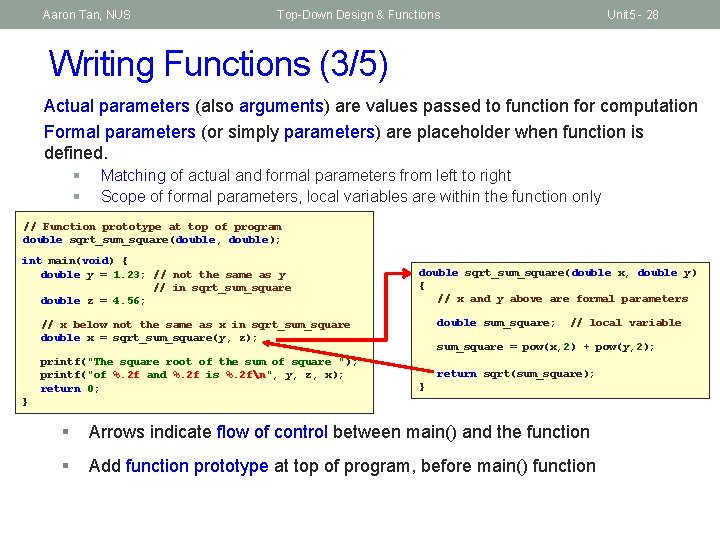Aaron Tan, NUS Top-Down Design & Functions Unit 5 - 28 Writing Functions (3/5) Actual parameters (also arguments) are values passed to function for computation Formal parameters (or simply parameters) are placeholder when function is defined. § § Matching of actual and formal parameters from left to right Scope of formal parameters, local variables are within the function only // Function prototype at top of program double sqrt_sum_square(double, double); int main(void) { double y = 1. 23; // not the same as y // in sqrt_sum_square double z = 4. 56; double sqrt_sum_square(double x, double y) { // x and y above are formal parameters double sum_square; // x below not the same as x in sqrt_sum_square double x = sqrt_sum_square(y, z); printf("The square root of the sum of square "); printf("of %. 2 f and %. 2 f is %. 2 fn", y, z, x); return 0; // local variable sum_square = pow(x, 2) + pow(y, 2); return sqrt(sum_square); } } § Arrows indicate flow of control between main() and the function § Add function prototype at top of program, before main() functionAaron Tan, NUS Top-Down Design & Functions Unit 5 - 29 Writing Functions (4/5) Unit 5_Function. Eg. c #include <stdio. h> #include <math. h> The complete program /* Function prototype placed at top of program */ double sqrt_sum_square(double, double); int main(void) { double y = 1. 23; double z = 4. 56; // not the same as y in sqrt_sum_square // x below has nothing to do with x in sqrt_sum_square double x = sqrt_sum_square( y, z ); // in the previous statement, y and z are actual parameters printf("The square root of the sum of squares " ); printf("of %. 2 f and %. 2 f is %. 2 fn", y, z, x); return 0; } /* Finds the square root of the * sum of the squares of the two parameters * Precond: x and y are non-negative numbers */ double sqrt_sum_square(double x, double y) { // x and y above are the formal parameters double sum_square; // local variable declaration sum_square = pow(x, 2) + pow(y, 2); return sqrt(sum_square); }Aaron Tan, NUS Top-Down Design & Functions Unit 5 - 30 Writing Functions (5/5) § Use of functions allow us to manage a complex (abstract) task with a number of simple (specific) ones. § This allows us to switch between abstract and go to specific at ease to eventually solve the problem. § Function allows a team of programmers working together on a large program – each programmer will be responsible for a particular set of functions. § Function is good mechanism to allow re-use across different programs. Programmers use functions like building blocks. § Function allows incremental implementation and testing (with the use of driver function to call the function and then to check the output) § Acronym NOT summarizes the requirements for argument list correspondence. (N: number of arguments, O: order, and T: type).Aaron Tan, NUS Top-Down Design & Functions Unit 5 - 31 Ex #1: A Simple “Drawing” Program (1/3) Problem: § Write a program Unit 5_Draw. Figures. c to draw a rocket ship (which is a triangle over a rectangle over an inverted V), a male stick figure (a circle over a rectangle over an inverted V), and a female stick figure (a circle over a triangle over an inverted V) rocket male Analysis: § No particular input needed, just draw the needed 3 figures § There are common shapes shared by the 3 figures Design: § Algorithm (in words): 1. Draw Rocket ship 2. Draw Male stick figure (below Rocket ship) 3. Draw Female stick figure (below Male stick figure) female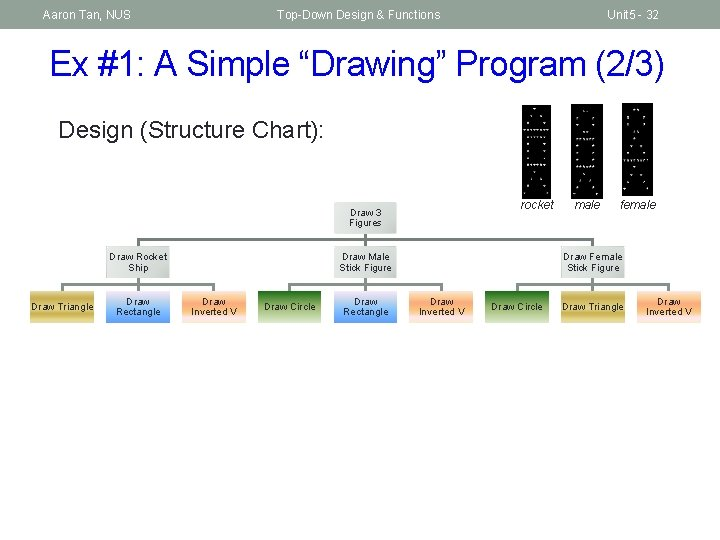Aaron Tan, NUS Top-Down Design & Functions Unit 5 - 32 Ex #1: A Simple “Drawing” Program (2/3) Design (Structure Chart): rocket Draw 3 Figures Draw Rocket Ship Draw Triangle Draw Rectangle Draw Male Stick Figure Draw Inverted V Draw Circle Draw Rectangle male female Draw Female Stick Figure Draw Inverted V Draw Circle Draw Triangle Draw Inverted V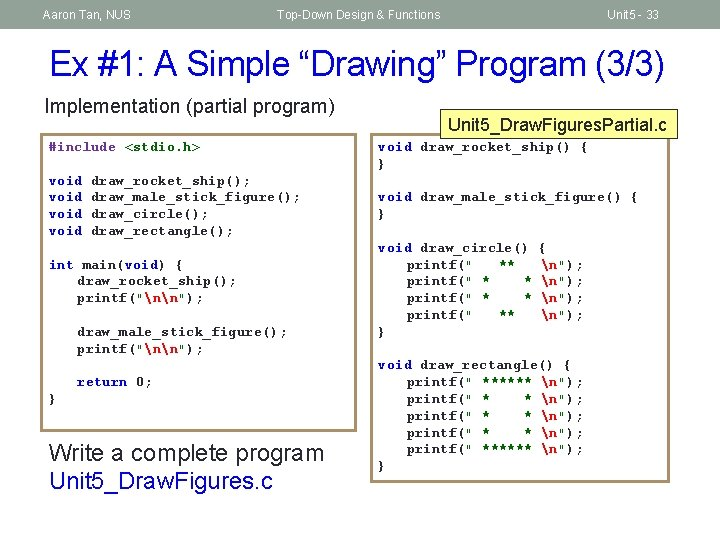Aaron Tan, NUS Top-Down Design & Functions Unit 5 - 33 Ex #1: A Simple “Drawing” Program (3/3) Implementation (partial program) #include <stdio. h> void draw_rocket_ship(); draw_male_stick_figure(); draw_circle(); draw_rectangle(); int main(void) { draw_rocket_ship(); printf("nn"); draw_male_stick_figure(); printf("nn"); return 0; } Write a complete program Unit 5_Draw. Figures. c Unit 5_Draw. Figures. Partial. c void draw_rocket_ship() { } void draw_male_stick_figure() { } void draw_circle() printf(" ** printf(" ** } { n"); void draw_rectangle() { printf(" ****** n"); printf(" * * n"); printf(" ****** n"); }Aaron Tan, NUS Top-Down Design & Functions Unit 5 - 34 Pass-By-Value and Scope Rules (1/4) § In C, the actual parameters are passed to the formal parameters by a mechanism known as pass-by-value. int main(void) { double a = 10. 5, b = 7. 8; printf("%. 2 fn", srqt_sum_square(3. 2, 12/5); printf("%. 2 fn", srqt_sum_square(a, a+b); return 0; a b 10. 5 7. 8 Actual parameters: 10. 5 3. 2 and 18. 3 2. 0 } double sqrt_sum_square(double x, double y) { double sum_square; sum_square = pow(x, 2) + pow(y, 2); return sqrt(sum_square); } Formal parameters: x y 10. 5 3. 2 18. 3 2. 0Aaron Tan, NUS Top-Down Design & Functions Unit 5 - 35 Pass-By-Value and Scope Rules (2/4) § Formal parameters are local to the function they are declared in. § Variables declared within the function are also local to the function. § Local parameters and variables are only accessible in the function they are declared – scope rule. § When a function is called, an activation record is created in the call stack, and memory is allocated for the local parameters and variables of the function. § Once the function is done, the activation record is removed, and memory allocated for the local parameters and variables is released. § Hence, local parameters and variables of a function exist in memory only during the execution of the function. They are called automatic variables. § In contrast, static variables exist in the memory even after the function is executed. (We will not use static variables in CS 1010. )Aaron Tan, NUS Top-Down Design & Functions Unit 5 - 36 Pass-By-Value and Scope Rules (3/4) § Spot the error in this code: int f(int); int main(void) { int a; . . . } int f(int x) { return a + x; }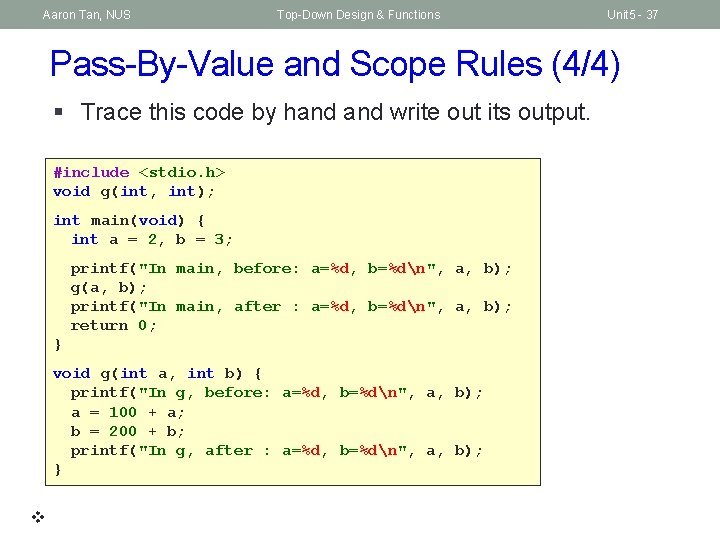Aaron Tan, NUS Top-Down Design & Functions Unit 5 - 37 Pass-By-Value and Scope Rules (4/4) § Trace this code by hand write out its output. #include <stdio. h> void g(int, int); int main(void) { int a = 2, b = 3; printf("In main, before: a=%d, b=%dn", a, b); g(a, b); printf("In main, after : a=%d, b=%dn", a, b); return 0; } void g(int a, int b) { printf("In g, before: a=%d, b=%dn", a, b); a = 100 + a; b = 200 + b; printf("In g, after : a=%d, b=%dn", a, b); }Aaron Tan, NUS Top-Down Design & Functions Unit 5 - 38 Consequence of Pass-By-Value § Can this code be used to swap the values in a and b? #include <stdio. h> void swap(int, int); int main(void) { int a = 2, b = 3; printf("In main, before: a=%d, b=%dn", a, b); swap(a, b); printf("In main, after : a=%d, b=%dn", a, b); return 0; } void swap(int a, int b) { int temp = a; a = b; b = temp; }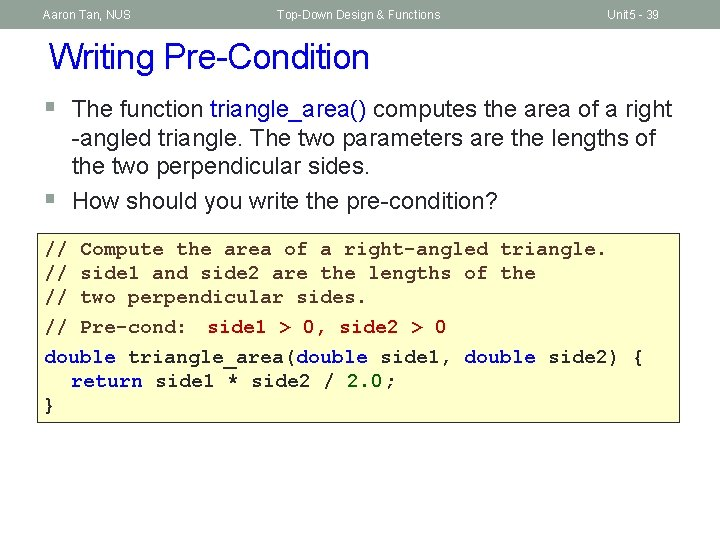Aaron Tan, NUS Top-Down Design & Functions Unit 5 - 39 Writing Pre-Condition § The function triangle_area() computes the area of a right § -angled triangle. The two parameters are the lengths of the two perpendicular sides. How should you write the pre-condition? // Compute the area of a right-angled triangle. // side 1 and side 2 are the lengths of the // two perpendicular sides. // Pre-cond: side 1 > 0, side 2 > 0 double triangle_area(double side 1, double side 2) { return side 1 * side 2 / 2. 0; }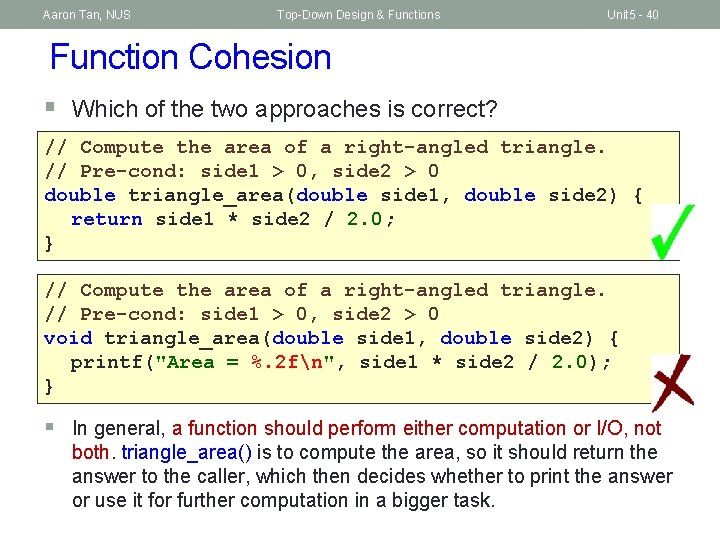Aaron Tan, NUS Top-Down Design & Functions Unit 5 - 40 Function Cohesion § Which of the two approaches is correct? // Compute the area of a right-angled triangle. // Pre-cond: side 1 > 0, side 2 > 0 double triangle_area(double side 1, double side 2) { return side 1 * side 2 / 2. 0; } // Compute the area of a right-angled triangle. // Pre-cond: side 1 > 0, side 2 > 0 void triangle_area(double side 1, double side 2) { printf("Area = %. 2 fn", side 1 * side 2 / 2. 0); } § In general, a function should perform either computation or I/O, not both. triangle_area() is to compute the area, so it should return the answer to the caller, which then decides whether to print the answer or use it for further computation in a bigger task.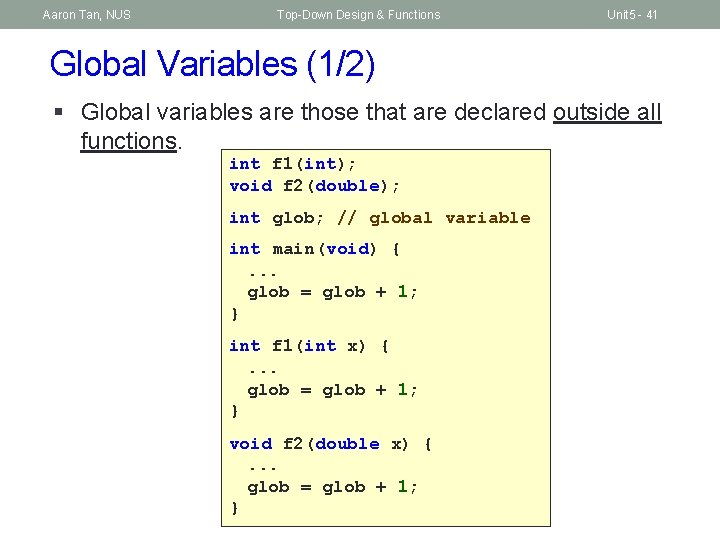Aaron Tan, NUS Top-Down Design & Functions Unit 5 - 41 Global Variables (1/2) § Global variables are those that are declared outside all functions. int f 1(int); void f 2(double); int glob; // global variable int main(void) {. . . glob = glob + 1; } int f 1(int x) {. . . glob = glob + 1; } void f 2(double x) {. . . glob = glob + 1; }Aaron Tan, NUS Top-Down Design & Functions Unit 5 - 42 Global Variables (2/2) § Global variables can be accessed and modified by any function! § Because of this, it is hard to trace when and where the global variables are modified. § Hence, we will NOT allow the use of global variables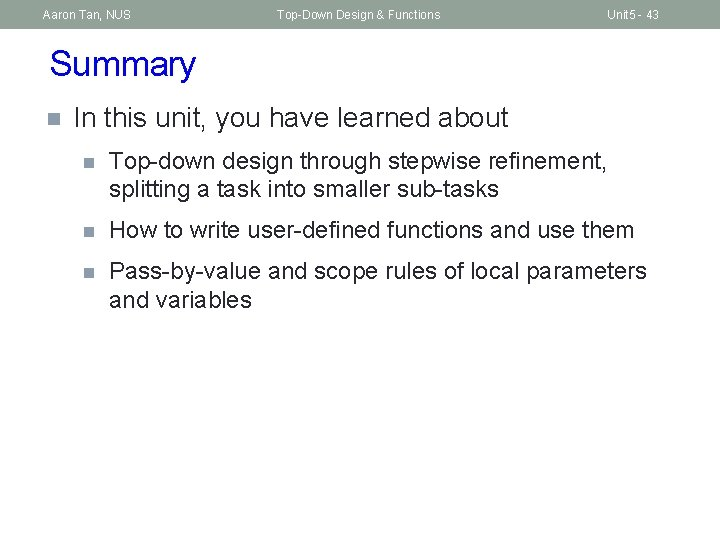Aaron Tan, NUS Top-Down Design & Functions Unit 5 - 43 Summary n In this unit, you have learned about n Top-down design through stepwise refinement, splitting a task into smaller sub-tasks n How to write user-defined functions and use them n Pass-by-value and scope rules of local parameters and variables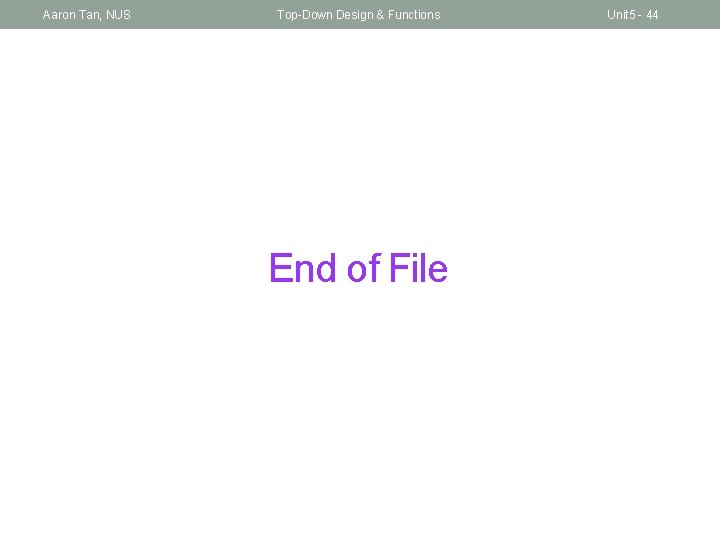Aaron Tan, NUS Top-Down Design & Functions End of File Unit 5 - 44